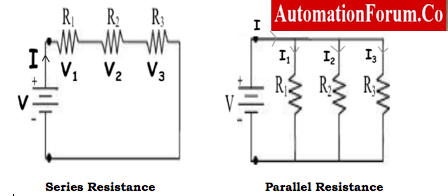# Simplifier Formulas for Series and Parallel Circuits Resistance Calculations## Combining resistors

• Resistors are in either fixed or variable range. It would be impracticable for manufacturers to build resistors in that form, they don’t exist at all.
• A 25k resistor, for instance, may be impossible to locate, whereas 22k resistors are widespread and are available almost anywhere.

### Series Resistance

When two resistors are connected in series, the current is first limited or restricted by the first resistor and then further limited or restricted by the second resistor, increasing the effect of the series connection.

#### Equivalent Series Resistance

The total resistor value in a series circuit connection is equal to R, Rseries, or Req or R effective.

R = R1+R2+ R3

#### Problem 1

Determine the value of equivalent resistance in the following circuit.

Req = 1200 + 680 + 470 Ω = 2350 Ω

### Current flow & Voltage drops across the series elements

• There is only one direction for the current, I, to move.
• As a result, each resistor is experiencing the same amount of current, I.
• Each resistor is experiencing the same amount of current, I.
• The potential difference, V, is directly proportional to resistance R.

I = I1= I2 = I3

V= V1+V2

The Vout in a given circuit is calculated as

### Simplified formula for same value resistance in series (R1 = R2 = R3)

• The total or equivalent resistance, RT, is equal to double the value of one resistor if two identical resistances or impedances are connected in series.
• That is equal to 2R, 3R, etc., for three identical resistors connected in series.

### Finding unknown resistor in circuit

• When total resistance is known, the unknown resistance value in circuit can be found by subtracting known resistor value from equivalent resistor of the circuit. ( Rx= Req – R1 )
• When applied voltage and current flow in circuit is known then Req is found by V/I. Same procedure mentioned above is followed to determine unknown resistance

#### Problem 2

If one of the resistors has a resistance of 2Ω. Determine the value of the other resistor when total resistance is 7Ω

Rx= Req – R1= 7 – 2 = 5 Ω

Rx= R2 = 5 Ω

## Parallel Resistance

• When a resistor is connected in parallel, two paths for current flow are created.
• Parallelism has the effect of lowering overall resistance.
• Equivalent Parallel Resistance

### The equivalent resistance.

The corresponding / effective resistance for 2 resistors in parallel

### When paralleling more than two or three resistors

The equivalent /effective resistance becomes

#### Problem 3

Two resistors with resistances of 3 and 6 are linked in parallel, which is a problem. Identify the overall resistance.

### Simplified formula for same value resistance in parallel (R1 = R2 = R3)

The total or equivalent resistance, RT, is equal to half the value of one resistor if the two parallel resistances or impedances are equal and of the same value. That is equivalent to R/2, R/3, etc., for three parallel resistors of identical value.

#### Problem 4

Determine the equivalent resistor in following circuit.

### Finding unknown resistance in parallel combination

#### Problem 5

What resistance value needs to be added in parallel to an 12 ohm resistor in circuit to produce a total resistance of 3 ohms?

R1 = 12 ohm & RT= 3 ohm

From above formula R2 = (12×3)/ (12 – 3) = 4 ohms

## Comparison of Series & Parallel Combination

Series Combination1. The circuit’s total current is the SAME throughout.
2. The bulbs now illuminate at the same brightness.
3. One switch is used to operate every component of the circuit.
1. Electric current can only go along one path.
2. Other bulbs won’t light up if a lamp or bulb is burned.
Series of cells do not endure very long.
3. As more bulbs are added, the circuit’s resistance rises and the amount of electric current flowing decreases.
Parallel
Combination
1. Even if one of the bulbs burns out, the others still shine.
2. Parallel cells are more durable.
3. The voltage delivered to each bulb by the power source is the same.
1. If cells of the same type are linked in parallel, the voltage does not rise or fall.
2. Even though more cells are added in parallel, the electric current in the circuit remains constant.